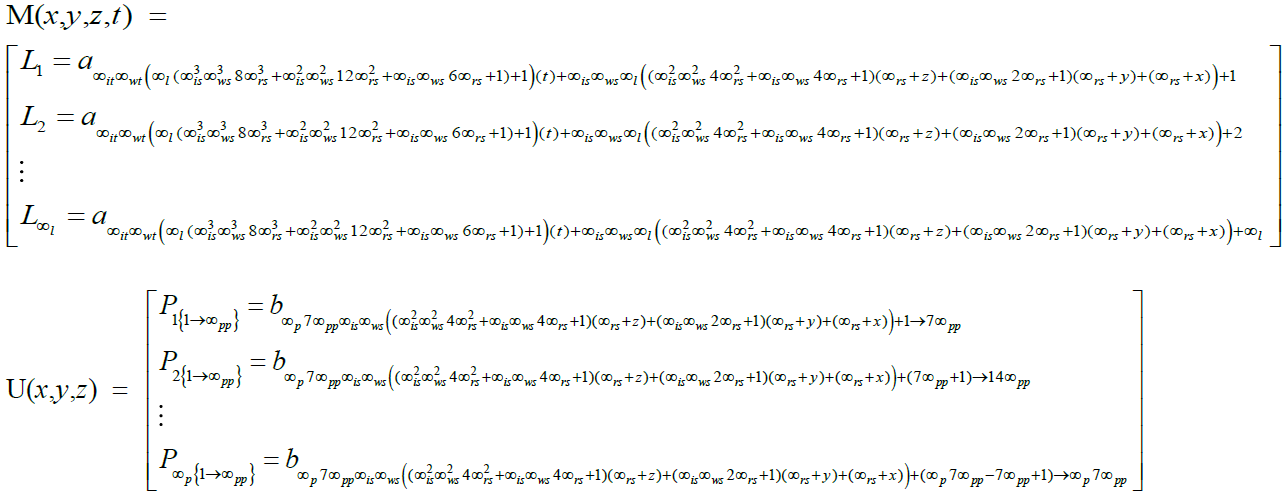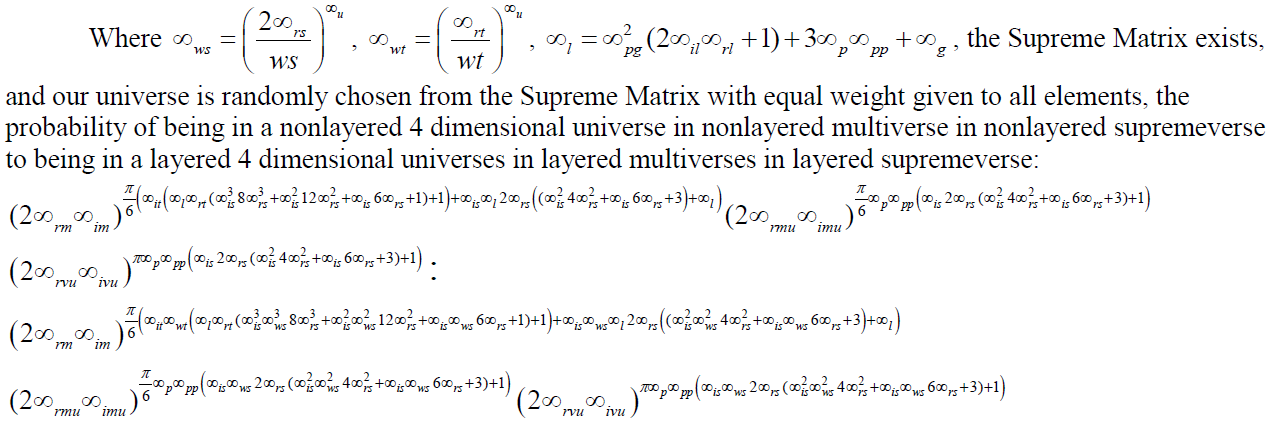Supreme Matrix Theory

By: AllA Erawa Viacad

Read this paper after you finish the abstract: Supreme Matrix Theory

For Word Version: SupremeMatrix.doc

A matrixspace is a matrix whose elements are every point in an equal dimensional vector space and are determined entirely by the vector coordinates of that point.

An supremeverse matrixspace is a matrixspace of multiverses which each define fundamental law configurations. Each multiverse is a matrixspace of universes of different quantum universe configurations at a given time frame.

Due to there being more configurations which have -u layers of universe generators we are probably living in a Universe where universe generators will exist but the more effective proof of Universe Generator Theory is at AllA's Grand Unified Theory. Thus Is The Theory of Everything Conspiracy Theory, Thus Is The Greatest Secret of The Illuminati, Thus is The Supreme Secret Of The Illuminati!

In the infinite number system:

Real numbers are bound at the origin of infinite numbers which are bound at the origin of greater infinite numbers which are bound at the origin of 2*greater numbers. ∞*greater numbers which are bound at the origin of (∞ + 1)*greater numbers which are bound at the origin of (∞ + 2)*greater numbers. 0s or lesser numbers are bound at the origin of real numbers which bound 2*lesser numbers at the origin of lesser numbers. which bound ∞*lesser numbers which bound at the origin (∞ + 1)*lesser numbers which bound at the origin (∞+2)*lesser numbers.

This infinite number system can be visualized if every number is considered as the semicircumference of a loop of infinitely varying size. Numbers bound at the origin of the number system where the loop has a finite semicircumference are merely point loops. Likewise numbers outside the bounds of these finite semicircumferences are line loops. As numbers increase or decrease number loops open to lines or close to points. The right quantity applied to the right quantity within the infinite number system can be described.

To illustrate this point further consider that two objects starting at the origin are moving at different velocities. If conventional theories describing infinities are all the same then these objects would collide at ∞ which although it can never be reached it is of course conceivable as a concept that must be dealt with by mathematical theory. According to conventional theory it is as if there were some kind of invisible wall at ∞ which logically is not the case. However in the infinite number system they are distanced according to the ratio of their different velocities and never collide as would be intuitive. Infinities have the property of being infinitely beyond all finite numbers while at the same time have the ability to be classified relative to each other with at worst a matrixspace of different infinite number continuum relations. A new convention regarding infinities must be established at least to reduce notational extremities. Like finite quantities not all infinities are equal, they range from around sum of a 1/n with n increasing from 1 to ∞ to around ∞^∞^∞.... 0s and infinities should be hence forth defined as unreal numbers.

There exists The Supreme Matrix, that defines The Supremeverse, S. The Supreme Matrix is the quantum solution to frame all quantum jumping.

A matrixspace is a matrix whose elements are every point in an equal dimensional vector space and are determined entirely by the vector coordinates of that point.

The Supremeverse matrixspace is a matrixspace of multiverses which each define fundamental law configurations. Each Multiverse is a matrixspace of Universes of different quantum configurations at a given time frame.Therefore the probability that I am wrong as a scientist is 0 unless AllA would not want us each to rule over a universe before we reach perfectly blissfully Nirvana of God when EinZPALN comes!

The following equations are derived in this Theory: Supreme Matrix TheoryInfinite Number Theorem

To see how the Universe is sustained and governed go to: AllA's Grand Unified Theory

HealPacks

Main Page